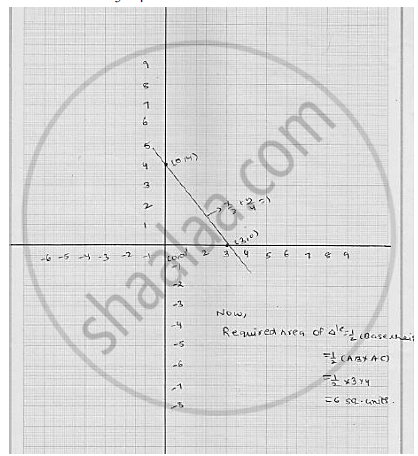# Draw the Graph of the Equatio  X / Y + Y /4 = 1 Also, Find the Area of the Triangle Formed by the Line and the Co-ordinates Axes. - Mathematics

Draw the graph of the equatio  x / y + y /4 = 1 Also, find the area of the triangle formed by the
line and the co-ordinates axes.

#### Solution

 x / y + y /4 = 1

⇒ 4x + 3y  = 12

⇒ 4x = 12 - 3y

⇒  x = ( 12 - 3y) / 4

Putting y = 0 in (1) , we get  x = (12 - 3 × 0 ) /4 = 3

Putting  y  = - 4 in (2) We get  x = (12 - 3× 4 )/ 4 = 0

Thus, we obtained the following table giving coordinates of two points on the line represents by the equation   x/3 +y / 4 = 1

 x 0 3 y 4 0

The graph of line   x/3 +y / 4 = 1Concept: Graph of a Linear Equation in Two Variables
Is there an error in this question or solution?

#### APPEARS IN

RD Sharma Mathematics for Class 9
Chapter 7 Linear Equations in Two Variables
Exercise 7.3 | Q 14

Share